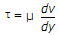# Civil Engineering - Hydraulics

### Exercise :: Hydraulics - Section 3

36.

Most economical section of a triangular channel, is

 A. equilateral triangle B. right angled triangle C. isosceles triangle with 45° vertex angle D. right angled triangle with equal sides.

Explanation:

No answer description available for this question. Let us discuss.

37.

In an open tube, free surface of mercury remains

 A. horizontal B. curved upwards C. curved downwards D. none of these.

Explanation:

No answer description available for this question. Let us discuss.

38.

The equationfor the viscosity, is suggested

 A. Bernoulli B. Newton C. Chezy D. Bezin E. Helmholtz.

Explanation:

No answer description available for this question. Let us discuss.

39.

The value of momentum correction factor (β) for a laminar flow through a circular pipe, is

 A. 1/2 B. 2/3 C. 3/4 D. 4/3 E. 3/2

Explanation:

No answer description available for this question. Let us discuss.

40.

Manometers are used to measure

 A. pressure in water channels, pipes etc. B. difference in pressure at two points C. atmospheric pressure D. very low pressure.